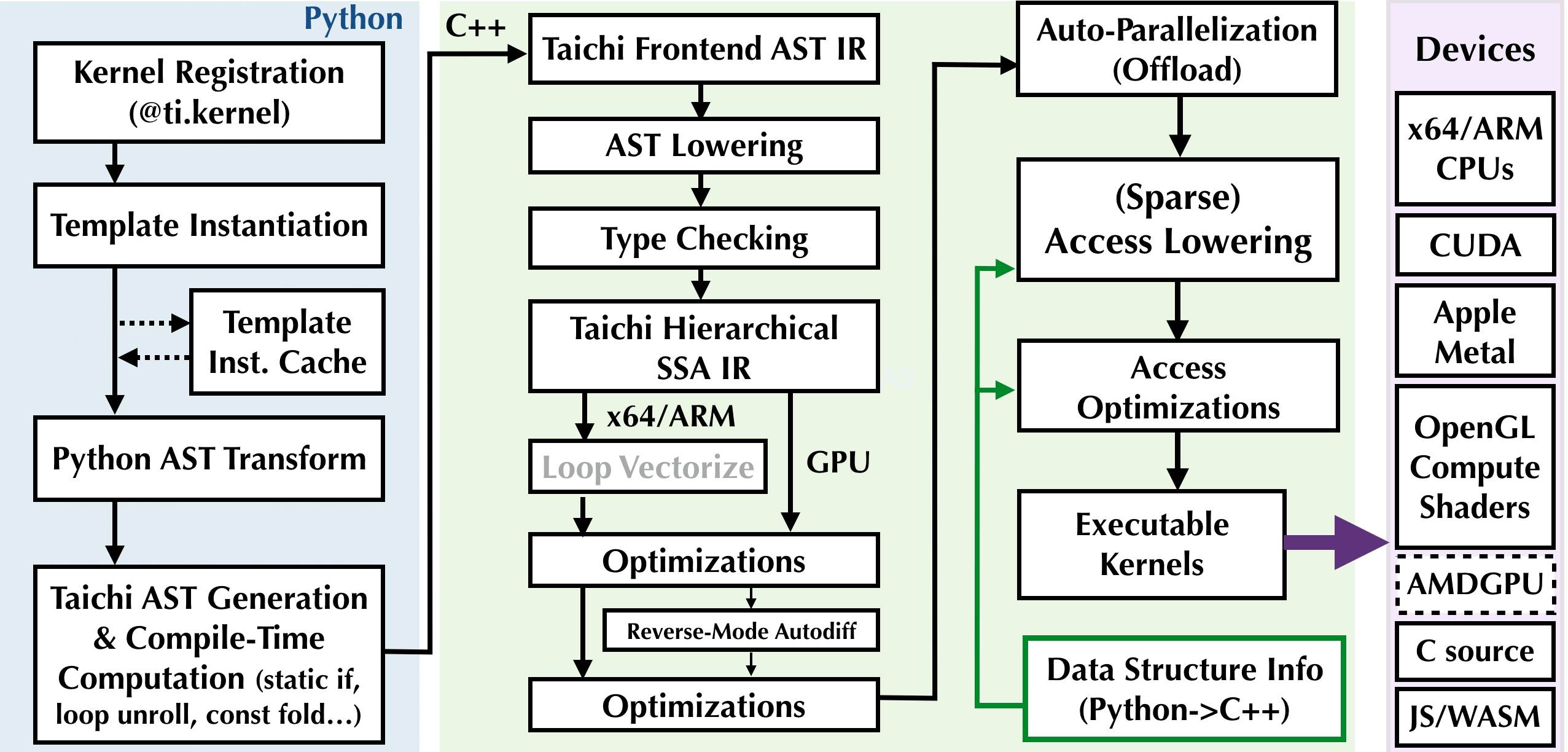Version: v1.6.0

# 一个 Taichi kernel 的生命周期

• 登记kernel
• 模板实例化及缓存
• Python AST转换
• Taichi IR的编译、优化及可执行生成
• 启动``@ti.kerneldef add(field: ti.template(), delta: ti.i32):    for i in field:        field[i] += delta``

``x = ti.field(dtype=ti.f32, shape=128)y = ti.field(dtype=ti.f32, shape=16)``

## 模板实例化及缓存

``add(x, 42)``

When `add` is called for the first time, the Taichi frontend compiler will instantiate the kernel.

When you have a second call with the same template signature (explained later), e.g.,

``add(x, 1)``

Taichi will directly reuse the previously compiled binary.

Arguments hinted with `ti.template()` are template arguments, and will incur template instantiation. 例如，

``add(y, 42)``

##### note

Template signatures are what distinguish different instantiations of a kernel template. The signature of `add(x, 42)` is `(x, ti.i32)`, which is the same as that of `add(x, 1)`. Therefore, the latter can reuse the previously compiled binary. The signature of `add(y, 42)` is `(y, ti.i32)`, a different value from the previous signature, hence a new kernel will be instantiated and compiled.

##### note

Many basic operations in the Taichi standard library are implemented using Taichi kernels using metaprogramming tricks. Invoking them will incur implicit kernel instantiations.

Examples include `x.to_numpy()` and `y.from_torch(torch_tensor)`. When you invoke these functions, you will see kernel instantiations, as Taichi kernels will be generated to offload the hard work to multiple CPU cores/GPUs.

As mentioned before, the second time you call the same operation, the cached compiled kernel will be reused and no further compilation is needed.

## Code transformation and optimizations

When a new instantiation happens, the Taichi frontend compiler (i.e., the `ASTTransformer` Python class) will transform the kernel body AST into a Python script, which, when executed, emits a Taichi frontend AST. Basically, some patches are applied to the Python AST so that the Taichi frontend can recognize it.

The Taichi AST lowering pass translates Taichi frontend IR into hierarchical static single assignment (SSA) IR, which allows a series of further IR passes to happen, such as

• Loop vectorization
• Type inference and checking
• General simplifications such as common subexpression elimination (CSE), dead instruction elimination (DIE), constant folding, and store forwarding
• Access lowering
• Data access optimizations
• Reverse-mode automatic differentiation (if using differentiable programming)